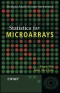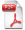# Details## Introduction to Biostatistical Applications in Health Research with Microsoft Office Excel and R

2. Aufl.
 von: Robert P. Hirsch 114,99 € Verlag: Wiley Format: EPUB Veröffentl.: 28.01.2021 ISBN/EAN: 9781119722649 Sprache: englisch Anzahl Seiten: 640

DRM-geschütztes eBook, Sie benötigen z.B. Adobe Digital Editions und eine Adobe ID zum Lesen.

## Beschreibungen

#### Titelbeschreibung

<p>The second edition of <i>Introduction to Biostatistical Applications in Health Research</i> delivers a thorough examination of the basic techniques and most commonly used statistical methods in health research. Retaining much of what was popular with the well-received first edition, the thoroughly revised second edition includes a new chapter on testing assumptions and how to evaluate whether those assumptions are satisfied and what to do if they are not.</p> <p>The newest edition contains brand-new code examples for using the popular computer language R to perform the statistical analyses described in the chapters within. You'll learn how to use Excel to generate datasets for R, which can then be used to conduct statistical calculations on your data.</p> <p>The book also includes a companion website with a new version of BAHR add-in programs for Excel. This new version contains new programs for nonparametric analyses, Student-Newman-Keuls tests, and stratified analyses. Readers will also benefit from coverage of topics like:</p> <ul> <li>Extensive discussions of basic and foundational concepts in statistical methods, including Bayes' Theorem, populations, and samples</li> <li>A treatment of univariable analysis, covering topics like continuous dependent variables and ordinal dependent variables</li> <li>An examination of bivariable analysis, including regression analysis and correlation analysis</li> <li>An analysis of multivariate calculations in statistics and how testing assumptions, like assuming Gaussian distributions or equal variances, affect statistical outcomes</li> </ul> <p>Perfect for health researchers of all kinds, Introduction to Biostatistical Applications in Health Research also belongs on the bookshelves of anyone who wishes to better understand health research literature. Even those without a great deal of mathematical background will benefit greatly from this text.</p>

#### Inhaltsverzeichnis

<p>Preface</p> <p>PART ONE:  Basic Concepts</p> <p>1.Thinking About Chance</p> <p>1.1.Properties of Chance</p> <p>1.2.Combinations of events</p> <p>1.2.1 Intersections</p> <p>1.2.2 Unions</p> <p>1.3.Bayes’ theorem</p> <p>2.Describing Populations</p> <p>2.1.Types of data</p> <p>2.2.Describing distributions graphically</p> <p>2.2.1.  Graphing discrete data</p> <p>2.2.2.  Graphing continuous data</p> <p>2.2.3.  Frequency polygon</p> <p>2.3.Describing distributions mathematically</p> <p>2.3.1.  Parameter of location</p> <p>2.3.2.  Parameter of dispersion</p> <p>2.4    Taking chance into account</p> <p>2.4.1 Standard normal distribution</p> <p>3.Examining Samples</p> <p>3.1.Nature of samples</p> <p>3.2.Estimation</p> <p>3.2.1 Point estimates</p> <p>3.2.2 The sampling distribution</p> <p>3.2.3 Interval estimates</p> <p>3.3.Hypothesis testing</p> <p>PART TWO:  Univariable Analysis</p> <p>4.Univariable Analysis of a Continuous Dependent Variable</p> <p>4.1.Student’s t distribution</p> <p>4.2.Interval estimation</p> <p>4.3.Hypothesis testing</p> <p>5.Univariable Analysis of an Ordinal Dependent Variable</p> <p>5.1  Nonparametric methods</p> <p>5.2  Estimation</p> <p>5.3   Wilcoxon signed-rank test</p> <p>5.4   Statistical power of nonparametric tests</p> <p>6.Univariable Analysis of a Nominal Dependent Variable</p> <p>6.1.Distributions of nominal data</p> <p>6.2.Point estimates</p> <p>6.2.1 Proportions</p> <p>6.2.2 Rates</p> <p>6.3.Sampling distributions</p> <p>6.3.1 Binomial distribution</p> <p>6.3.2 Poisson distribution</p> <p>6.4.Interval estimation</p> <p>6.5.Hypothesis testing</p> <p>PART THREE:  Bivariable Analysis</p> <p>7.Bivariable Analysis of a Continuous Dependent Variable</p> <p>7.1.Continuous independent variable</p> <p>7.1.1 Regression analysis</p> <p>7.1.2 Correlation analysis</p> <p>7.2.Ordinal independent variable</p> <p>7.3.Nominal independent variable</p> <p>7.3.1 Estimating the difference between groups</p> <p>7.3.2 Taking chance into account</p> <p>8.Bivariable Analysis of an Ordinal Dependent Variable</p> <p>8.1.Ordinal independent variable</p> <p>8.2.Nominal independent variable</p> <p>9.Bivariable Analysis of a Nominal Dependent Variable</p> <p>9.1.Continuous independent variable</p> <p>9.1.1 Estimation</p> <p>9.1.2 Hypothesis testing</p> <p>9.2.Nominal independent variable</p> <p>9.2.1 Dependent variable not affected by time:  Unpaired design</p> <p>9.2.2 Dependent variable not affected by time:  Paired design</p> <p>9.2.3 Dependent variable affected by time</p> <p>PART FOUR:  Multivariable Analysis</p> <p>10.Multivariable Analysis of a Continuous Dependent Variable</p> <p>10.1.Continuous independent variables</p> <p>10.1.1 Multiple regression analysis</p> <p>10.1.2 Multiple correlation analysis</p> <p>10.2.Nominal independent variables</p> <p>10.2.1 Analysis of variance</p> <p>10.2.2 Posterior tests</p> <p>10.3.Continuous and nominal independent variables</p> <p>10.3.1 Indicator (“dummy”) variables</p> <p>10.3.2 Interaction variables</p> <p>10.3.3 General linear model</p> <p>11.Multivariable Analysis of an Ordinal Dependent Variable</p> <p>11.1.Nonparametric ANOVA</p> <p>11.2.Posterior testing</p> <p>12.Multivariable Analysis of a Nominal Dependent Variable</p> <p>12.1.Continuous and/or nominal independent variables</p> <p>12.1.1 Maximum likelihood estimation</p> <p>12.1.2 Logistic regression analysis</p> <p>12.1.3 Cox regression analysis</p> <p>12.2.Nominal independent variables</p> <p>12.2.1 Stratified analysis</p> <p>12.2.2 Life table analysis</p> <p>13. Testing Assumptions</p> <p>13.1Continuous dependent variables</p> <p>13.1.1   Assuming a Gaussian distribution</p> <p>13.1.2   Transforming dependent variables</p> <p>13.1.3   Assuming equal variances</p> <p>13.1.4   Assuming additive relationships</p> <p>13.2Nominal dependent variables</p> <p>13.2.1   Assuming a Gaussian distribution</p> <p>13.2.2   Assuming equal variances</p> <p>13.2.3   Assuming additive relationships</p> <p>13.3Independent variables</p>

#### Rezension

"This book provides a good introduction to biostatistics with a lot of medical examples and exercises. It is perfect for those that have basic notions on mathematics, explaining the main formulas necessary for describing, testing and finding out the relationships between data ... the manuscript is very good, comprehensive in information, the chapters are well structured, it includes a great arsenal of examples analysed and described in the field of biostatistics at a basic level. The reader should achieve a solid first step knowledge in the area, both for the statistical concepts and also practical applications." <b>– International Society for Clinical Biostatistics News</b>

#### Autorenportrait

<p><b>ROBERT P. HIRSCH, PHD,</b> is on the faculty at the Foundation for Advanced Education in the Sciences as well as a Medical Research Consultant with over thirty years of experience. He received his doctorate in Biology at Kansas State University. He was formerly Professor at the George Washington University - Columbian College of Arts & Science where he helped to develop the Epidemiology and Biostatistics Programs.</p>

#### Back cover copy

<p><b>Learn more about the foundations of statistical methods in health research with this authoritative new resource</b></p> <p>The second edition of <i>Introduction to Biostatistical Applications in Health Research</i> delivers a thorough examination of the basic techniques and most commonly used statistical methods in health research. Retaining much of what was popular with the well-received first edition, the thoroughly revised second edition includes a new chapter on testing assumptions and how to evaluate whether those assumptions are satisfied and what to do if they are not.</p> <p>The newest edition contains brand-new code examples for using the popular computer language R to perform the statistical analyses described in the chapters within. You'll learn how to use Excel to generate datasets for R, which can then be used to conduct statistical calculations on your data.</p> <p>The book also includes a companion website with a new version of BAHR add-in programs for Excel. This new version contains new programs for nonparametric analyses, Student-Newman-Keuls tests, and stratified analyses. Readers will also benefit from coverage of topics like:</p> <ul> <li>Extensive discussions of basic and foundational concepts in statistical methods, including Bayes??? Theorem, populations, and samples</li> <li>A treatment of univariable analysis, covering topics like continuous dependent variables and ordinal dependent variables</li> <li>An examination of bivariable analysis, including regression analysis and correlation analysis</li> <li>An analysis of multivariate calculations in statistics and how testing assumptions, like assuming Gaussian distributions or equal variances, affect statistical outcomes</li> </ul> <p>Perfect for health researchers of all kinds, <i>Introduction to Biostatistical Applications in Health Research</i> also belongs on the bookshelves of anyone who wishes to better understand health research literature. Even those without a great deal of mathematical background will benefit greatly from this text.</p>

## Diese Produkte könnten Sie auch interessieren:Statistics for Microarrays
von: Ernst Wit, John McClure84,99 €